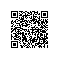# Python数据处理库pandas入门教程

pandas是一个Python语言的软件包，在我们使用Python语言进行机器学习编程的时候，这是一个非常常用的基础编程库。本文是对它的一个入门教程。

pandas提供了快速，灵活和富有表现力的数据结构，目的是使“关系”或“标记”数据的工作既简单又直观。它旨在成为在Python中进行实际数据分析的高级构建块。

# 入门介绍

pandas适合于许多不同类型的数据，包括：

• 具有异构类型列的表格数据，例如SQL表格或Excel数据
• 有序和无序（不一定是固定频率）时间序列数据。
• 具有行列标签的任意矩阵数据（均匀类型或不同类型）
• 任何其他形式的观测/统计数据集。

sudo pip3 install pandas

conda install pandas

# 核心数据结构

pandas最核心的就是SeriesDataFrame两个数据结构。

Series 1维 带有标签的同构类型数组
DataFrame 2维 表格结构，带有标签，大小可变，且可以包含异构的数据列

DataFrame可以看做是Series的容器，即：一个DataFrame中可以包含若干个Series。

## Series

# data_structure.py

import pandas as pd
import numpy as np

series1 = pd.Series([1, 2, 3, 4])
print("series1:\n{}\n".format(series1))

series1:
0    1
1    2
2    3
3    4
dtype: int64

• 输出的最后一行是Series中数据的类型，这里的数据都是int64类型的。
• 数据在第二列输出，第一列是数据的索引，在pandas中称之为Index

# data_structure.py

print("series1.values: {}\n".format(series1.values))

print("series1.index: {}\n".format(series1.index))

series1.values: [1 2 3 4]

series1.index: RangeIndex(start=0, stop=4, step=1)

# data_structure.py

series2 = pd.Series([1, 2, 3, 4, 5, 6, 7],
index=["C", "D", "E", "F", "G", "A", "B"])
print("series2:\n{}\n".format(series2))
print("E is {}\n".format(series2["E"]))

series2:
C    1
D    2
E    3
F    4
G    5
A    6
B    7
dtype: int64

E is 3

## DataFrame

# data_structure.py

df1 = pd.DataFrame(np.arange(16).reshape(4,4))
print("df1:\n{}\n".format(df1))

df1:
0   1   2   3
0   0   1   2   3
1   4   5   6   7
2   8   9  10  11
3  12  13  14  15

# data_structure.py

df2 = pd.DataFrame(np.arange(16).reshape(4,4),
columns=["column1", "column2", "column3", "column4"],
index=["a", "b", "c", "d"])
print("df2:\n{}\n".format(df2))

df2:
column1  column2  column3  column4
a        0        1        2        3
b        4        5        6        7
c        8        9       10       11
d       12       13       14       15

# data_structure.py

df3 = pd.DataFrame({"note" : ["C", "D", "E", "F", "G", "A", "B"],
"weekday": ["Mon", "Tue", "Wed", "Thu", "Fri", "Sat", "Sun"]})
print("df3:\n{}\n".format(df3))

df3:
note weekday
0    C     Mon
1    D     Tue
2    E     Wed
3    F     Thu
4    G     Fri
5    A     Sat
6    B     Sun

• DataFrame的不同列可以是不同的数据类型
• 如果以Series数组来创建DataFrame，每个Series将成为一行，而不是一列

# data_structure.py

noteSeries = pd.Series(["C", "D", "E", "F", "G", "A", "B"],
index=[1, 2, 3, 4, 5, 6, 7])
weekdaySeries = pd.Series(["Mon", "Tue", "Wed", "Thu", "Fri", "Sat", "Sun"],
index=[1, 2, 3, 4, 5, 6, 7])
df4 = pd.DataFrame([noteSeries, weekdaySeries])
print("df4:\n{}\n".format(df4))

df4的输出如下：

df4:
1    2    3    4    5    6    7
0    C    D    E    F    G    A    B
1  Mon  Tue  Wed  Thu  Fri  Sat  Sun

# data_structure.py

df3["No."] = pd.Series([1, 2, 3, 4, 5, 6, 7])
print("df3:\n{}\n".format(df3))

del df3["weekday"]
print("df3:\n{}\n".format(df3))

df3:
note weekday  No.
0    C     Mon    1
1    D     Tue    2
2    E     Wed    3
3    F     Thu    4
4    G     Fri    5
5    A     Sat    6
6    B     Sun    7

df3:
note  No.
0    C    1
1    D    2
2    E    3
3    F    4
4    G    5
5    A    6
6    B    7

## Index对象与数据访问

pandas的Index对象包含了描述轴的元数据信息。当创建Series或者DataFrame的时候，标签的数组或者序列会被转换成Index。可以通过下面的方式获取到DataFrame的列和行的Index对象：

# data_structure.py

print("df3.columns\n{}\n".format(df3.columns))
print("df3.index\n{}\n".format(df3.index))

df3.columns
Index(['note', 'No.'], dtype='object')

df3.index
RangeIndex(start=0, stop=7, step=1)

• Index并非集合，因此其中可以包含重复的数据
• Index对象的值是不可以改变，因此可以通过它安全的访问数据

DataFrame提供了下面两个操作符来访问其中的数据：

• loc：通过行和列的索引来访问数据
• iloc：通过行和列的下标来访问数据

# data_structure.py

print("Note C, D is:\n{}\n".format(df3.loc[[0, 1], "note"]))
print("Note C, D is:\n{}\n".format(df3.iloc[[0, 1], 0]))

Note C, D is:
0    C
1    D
Name: note, dtype: object

Note C, D is:
0    C
1    D
Name: note, dtype: object

# 文件操作

pandas库提供了一系列的read_函数来读取各种格式的文件，它们如下所示：

## 读取Excel文件

sudo pip3 install xlrd

$pip3 show xlrd Name: xlrd Version: 1.1.0 Summary: Library for developers to extract data from Microsoft Excel (tm) spreadsheet files Home-page: http://www.python-excel.org/ Author: John Machin Author-email: sjmachin@lexicon.net License: BSD Location: /Library/Frameworks/Python.framework/Versions/3.6/lib/python3.6/site-packages Requires:  接下来我们看一个读取Excel的简单的例子： # file_operation.py import pandas as pd import numpy as np df1 = pd.read_excel("data/test.xlsx") print("df1:\n{}\n".format(df1)) 这个Excel的内容如下： df1: C Mon 0 D Tue 1 E Wed 2 F Thu 3 G Fri 4 A Sat 5 B Sun 注：本文的代码和数据文件可以通过文章开头提到的Github仓库获取。 ## 读取CSV文件 下面，我们再来看读取CSV文件的例子。 第一个CSV文件内容如下： $ cat test1.csv
C,Mon
D,Tue
E,Wed
F,Thu
G,Fri
A,Sat

# file_operation.py

print("df2:\n{}\n".format(df2))

\$ cat test2.csv
C|Mon
D|Tue
E|Wed
F|Thu
G|Fri
A|Sat

# file_operation.py

print("df3:\n{}\n".format(df3))

path 文件路径
sep或者delimiter 字段分隔符
index_col 列号或名称用作结果中的行索引
names 结果的列名称列表
skiprows 从起始位置跳过的行数
na_values 代替NA的值序列
comment 以行结尾分隔注释的字符
parse_dates 尝试将数据解析为datetime。默认为False
keep_date_col 如果将列连接到解析日期，保留连接的列。默认为False
converters 列的转换器
dayfirst 当解析可以造成歧义的日期时，以内部形式存储。默认为False
data_parser 用来解析日期的函数
nrows 从文件开始读取的行数
iterator 返回一个TextParser对象，用于读取部分内容
chunksize 指定读取块的大小
skip_footer 文件末尾需要忽略的行数
verbose 输出各种解析输出的信息
encoding 文件编码
squeeze 如果解析的数据只包含一列，则返回一个Series
thousands 千数量的分隔符

# 处理无效值

# process_na.py

import pandas as pd
import numpy as np

df = pd.DataFrame([[1.0, np.nan, 3.0, 4.0],
[5.0, np.nan, np.nan, 8.0],
[9.0, np.nan, np.nan, 12.0],
[13.0, np.nan, 15.0, 16.0]])

print("df:\n{}\n".format(df));
print("df:\n{}\n".format(pd.isna(df)));****

df:
0   1     2     3
0   1.0 NaN   3.0   4.0
1   5.0 NaN   NaN   8.0
2   9.0 NaN   NaN  12.0
3  13.0 NaN  15.0  16.0

df:
0     1      2      3
0  False  True  False  False
1  False  True   True  False
2  False  True   True  False
3  False  True  False  False

## 忽略无效值

# process_na.py

print("df.dropna():\n{}\n".format(df.dropna()));

df.dropna():
Empty DataFrame
Columns: [0, 1, 2, 3]
Index: []

# process_na.py

print("df.dropna(axis=1, how='all'):\n{}\n".format(df.dropna(axis=1, how='all')));

df.dropna(axis=1, how='all'):
0     2     3
0   1.0   3.0   4.0
1   5.0   NaN   8.0
2   9.0   NaN  12.0
3  13.0  15.0  16.0

## 替换无效值

# process_na.py

print("df.fillna(1):\n{}\n".format(df.fillna(1)));

df.fillna(1):
0    1     2     3
0   1.0  1.0   3.0   4.0
1   5.0  1.0   1.0   8.0
2   9.0  1.0   1.0  12.0
3  13.0  1.0  15.0  16.0

# process_na.py

df.rename(index={0: 'index1', 1: 'index2', 2: 'index3', 3: 'index4'},
columns={0: 'col1', 1: 'col2', 2: 'col3', 3: 'col4'},
inplace=True);
df.fillna(value={'col2': 2}, inplace=True)
df.fillna(value={'col3': 7}, inplace=True)
print("df:\n{}\n".format(df));

df:
col1  col2  col3  col4
index1   1.0   2.0   3.0   4.0
index2   5.0   2.0   7.0   8.0
index3   9.0   2.0   7.0  12.0
index4  13.0   2.0  15.0  16.0

# 处理字符串

Seriesstr字段包含了一系列的函数用来处理字符串。并且，这些函数会自动处理无效值。

# process_string.py

import pandas as pd

s1 = pd.Series([' 1', '2 ', ' 3 ', '4', '5']);
print("s1.str.rstrip():\n{}\n".format(s1.str.lstrip()))
print("s1.str.strip():\n{}\n".format(s1.str.strip()))
print("s1.str.isdigit():\n{}\n".format(s1.str.isdigit()))

s1.str.rstrip():
0     1
1    2
2    3
3     4
4     5
dtype: object

s1.str.strip():
0    1
1    2
2    3
3    4
4    5
dtype: object

s1.str.isdigit():
0    False
1    False
2    False
3     True
4     True
dtype: bool

# process_string.py

s2 = pd.Series(['Stairway to Heaven', 'Eruption', 'Freebird',
'Comfortably Numb', 'All Along the Watchtower'])
print("s2.str.lower():\n{}\n".format(s2.str.lower()))
print("s2.str.upper():\n{}\n".format(s2.str.upper()))
print("s2.str.len():\n{}\n".format(s2.str.len()))

s2.str.lower():
0          stairway to heaven
1                    eruption
2                    freebird
3            comfortably numb
4    all along the watchtower
dtype: object

s2.str.upper():
0          STAIRWAY TO HEAVEN
1                    ERUPTION
2                    FREEBIRD
3            COMFORTABLY NUMB
4    ALL ALONG THE WATCHTOWER
dtype: object

s2.str.len():
0    18
1     8
2     8
3    16
4    24
dtype: int64

# 参考资料与推荐读物使用钉钉扫一扫加入圈子
+ 订阅